# Five Futoshiki Solving Strategies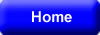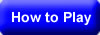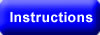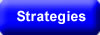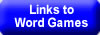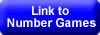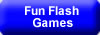Like sudoku, there are many techniques in solving a futoshiki puzzle. When the simple techniques don't work, you will need to use a more complicated solving technique. This can get to a point where it almost looks impossible to solve and may take a long time using a variety of strategies. But keep in mind, just like Sudoku and Kakuro, a Futoshiki puzzle has only one unique solution that will work successfully. In this article I will give you a few futoshiki strategies.

Strategy #1: Use Pencil marks

This is by far the first (and in my opinion most important) strategy, using pencil marks! Using pencil marks not only greatly reduce the chance for error, it helps to point out obvious hints in solving puzzles. Now, it seems like I am stating the obvious but it very easy to overlook it and think "I got it, it is no big deal" only to run into a problem later on. Using pencil marks, it is best to fill in possible values that you know are going to exist in a particular box. You don't need to over do it sometimes but it is good for a small range. Then, as more clues are revealed, you can reduce the pencil marks until only one digit remain and that will be the digit used for that box.

Strategy #2: Look for chains or a groups of chains

A good place to start a futoshiki puzzle is to look for chains. A chain is several connected boxes that are linked by the same "greater than" or "less than" sign. They don't necessarily need to be in any specific order just as long they are pointing in a specific direction. In a 6x6 puzzle Example A you can see a chain and thus you now know definitely what the values af the boxes are. In a 7x7 puzzle Example B you can see a chain but don't know exactly what values they are but you can deduce that it is a range of values.

 Example A (6x6)Example B (7x7)Example C (7x7)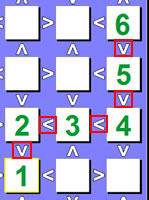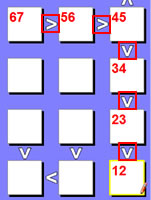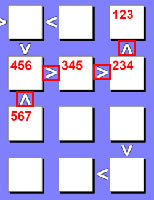This is a very good strategy for sudoku, kakuro and futoshiki puzzles assuming you are using pencil marks. If a box of a given row (or column) only has a pencil mark of 1 and 5 and a box in the same row (or column) also has a pencil mark of 1 and 5 then you can remove 1 and 5 from all other boxes in that row (or column). The reason behind this is that the first box must be a 1 or a 5 and the second box must be a 1 or a 5. If the 3rd box was set as a 5 for example, it would cause an obvious error in the row since you are not allowed to have the same digit in a row or column.Example Pair (5x5 puzzle)Example Triple (6x6 puzzle)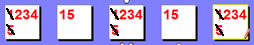��

Strategy #4: Lows and Highs

The unique thing about a futoshiki puzzle is that it is based off of inqeuality signs. This opens up the door to look for the high and low numbers to start off a puzzle. You can do this by looking for only one box in any given row or column that has all inequality signs either pointing in (for low) or pointing out (for high). Then you can determine exactly what that number is without any further guesswork. In the Example Low you can only put a 1 here because anywhere else if you put a 1 a "greater than" sign would mean that the adjacent box would be a zero. In the Example High you can only put a 6 here because anywhere else if you put a 6 a "less than" sign would mean that the adjacent box would be a seven and that is invalid in a 6x6 puzzle.Example Low (6x6 puzzle)Example High (6x6 puzzle)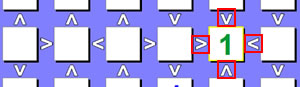��

Keep in mind that this strategy will only work if only one box in a row or column looks like this. If more than one row or column has this than there is more possibilities that either one could be a 1 or 6.

Strategy #5: Testing Digits (or Pencil-marks)

Here is one more final strategy for you which can be quite useful. It is using the "what if this box was a specific #?" scenario -or- "I know this box is a 1,4, or 5 so if I put a 4 here would I run into a snag?" From there, you can see if other digits will work out. If you find a snag in the end then you can eliminate that specific possibility (or pencil-mark) from that box to begin with.

 Example A (7x7 puzzle)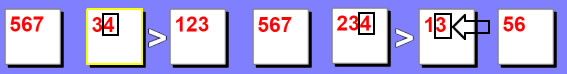In this example we will test the 3 in the 6th box. If we set the 6th box to 3, then that would force the 5th box to a 4 because 4 > 3. It will also set the 2nd box to 4 because it can't be a 3. This would make the 2nd box and the 5th box both as a 4 and that is not allowed so therefore the 3 can be removed as a possibility from box six.
 Example B (7x7 puzzle)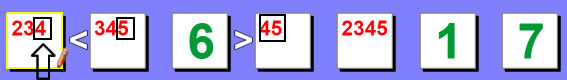In this example we will test the 4 in the first box. If we set the first box to 4, then that would force the 2nd box to a 5 because 4 < 5. It will also set the 4th box to 5 as well because it can't be a 4. You run into a problem where 2 boxes are a 5 in the same row and that is not allowed. So therefore, we can remove 4 as a possibility
 Example C (7x7 puzzle)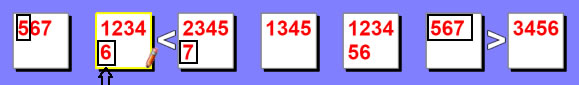In this example we will test the 6 in the 2nd box. If we set the 2nd box to 6, then that would force the 3rd box to a 7 because 6 < 7. It will also set the 1st box to 5 as well because it can't be a 6 or a 7. So now between the 1st, 2nd and 3rd boxes you have 5, 6 and 7. But this presents a problem with the 6th box which only allows 5, 6 or 7 to be used. As a result this would cause a duplicate value in the same row (or column), so we can remove 6 as a possibility from the 2nd box.
 Example D (7x7 puzzle)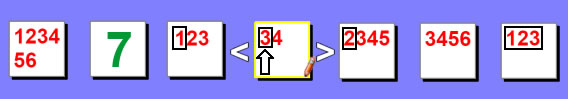In this example we will test the 3 in the 4th box. If we set the 4th box to 3, then that would force the 5th box to a 2 because 3 > 2. It will also force the 3rd box to a 1 as well because of the inequality rules and because we already used a 2. So now between the 3rd, 4th and 5th boxes you have 1, 3 and 2 respectively. But this presents a problem with the 7th box which only allows 1, 2 or 3 to be used. As a result this would cause a duplicate value in the same row (or column), so we can remove 3 as a possibility from the 4th box.
 Example E (7x7 puzzle) - Tricky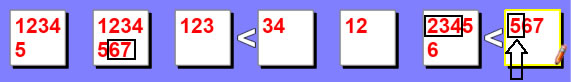In this example we will test the 5 in the 7th box. If we set the 7th box to 5, then that would force the 6th box to a 2, 3, or 4 because 2,3,4 < 5. This will create a situation in Box #2 since it is now the only box that has a 6 or 7. In the rules of a 7x7 futoshiki, #'s 1 through 7 must be used uniquely in each box across a row (or column). Given in this situation with the 6 and 7 only in Box #2, that would not be possible. So as a result, we can remove the 5 from the 7th box as a possibility.
 Example F (7x7 puzzle) - Tricky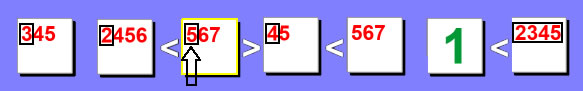This is a tricky example in which we will test the 5 in the 3rd box. If we set the 3rd box to 5, then that would force the 4th box to a 4. Then the 2nd box would have no choice but to be a 2 because 4 is used and 5, and 6 would violate the "less than" symbol. Having a 2, 5 and 4 on the board would now force the 1st box to a 3. So the numbers in order starting from box #1 would be 3, 2, 5, 4. Well now if you look at the possibilities in box #7, it is already a 2, 3, 4, 5 so no matter which one you choose, it would create a duplicate value for the entire row. So therefore, we can eliminate 5 as a possibility from box #3. Sometime you have to dig a little bit to reach this conclusion which can make it more tricky.
Good luck on your next Futoshiki puzzle!

Play: sudoku styles / kakuro / cryptoquote / online games / software design maintained by atksolutions.com